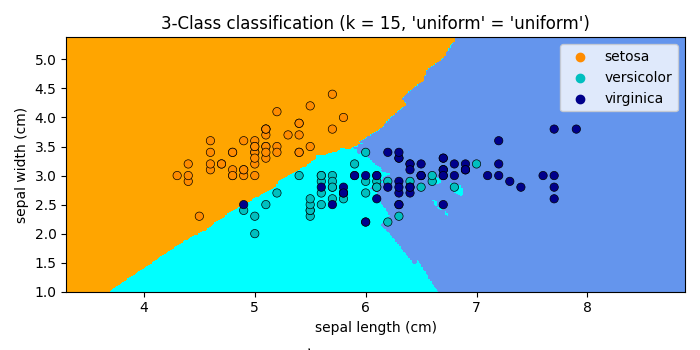# Graph k-NN decision boundaries in Matplotlib

MatplotlibPythonData Visualization

#### Python Data Science basics with Numpy, Pandas and Matplotlib

Most Popular

63 Lectures 6 hours

#### Data Visualization using MatPlotLib & Seaborn

11 Lectures 4 hours

#### MatPlotLib with Python

9 Lectures 2.5 hours

To make graph k-NN decision boundaries in matplotlib, we can take the following Steps.

## Steps

• Set the figure size and adjust the padding between and around the subplots.

• Initialize a variable n_neighbors for number of neighbors.

• Load and return the iris dataset (classification).

• Create x and y data points.

• Make lists of dark and light colors.

• Classifier implementing the k-nearest neighbors vote.

• Create xmin, xmax, ymin and ymax  data points.

• Create a new figure or activate an existing figure.

• Create a contourf plot.

• Create a scatter plot with X dataset.

• Set x and y axes labels, titles and scale of the axes.

• To display the figure, use Show() method.

## Example

import numpy as np
import matplotlib.pyplot as plt
import seaborn as sns
from matplotlib.colors import ListedColormap
from sklearn import neighbors, datasets

plt.rcParams["figure.figsize"] = [7.00, 3.50]
plt.rcParams["figure.autolayout"] = True

n_neighbors = 15
X = iris.data[:, :2]
y = iris.target
h = .02

cmap_light = ListedColormap(['orange', 'cyan', 'cornflowerblue'])
cmap_bold = ['darkorange', 'c', 'darkblue']

clf = neighbors.KNeighborsClassifier(n_neighbors, weights='uniform')
clf.fit(X, y)

x_min, x_max = X[:, 0].min() - 1, X[:, 0].max() + 1
y_min, y_max = X[:, 1].min() - 1, X[:, 1].max() + 1
xx, yy = np.meshgrid(np.arange(x_min, x_max, h),
np.arange(y_min, y_max, h))
Z = clf.predict(np.c_[xx.ravel(), yy.ravel()])
Z = Z.reshape(xx.shape)

plt.figure()

plt.contourf(xx, yy, Z, cmap=cmap_light)

sns.scatterplot(x=X[:, 0], y=X[:, 1], hue=iris.target_names[y],
palette=cmap_bold, alpha=1.0, edgecolor="black")

plt.xlim(xx.min(), xx.max())
plt.ylim(yy.min(), yy.max())

plt.title("3-Class classification (k = %i, 'uniform' = '%s')"
% (n_neighbors, 'uniform'))

plt.xlabel(iris.feature_names)
plt.ylabel(iris.feature_names)

plt.Show()

## Output

It will produce the following output −